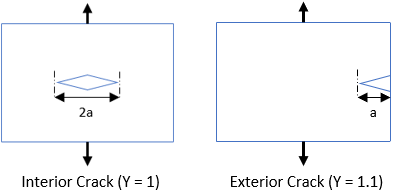## Critical Crack Length

Consider a part is formed by bending a sheet of 4340 Steel, which has a Fracture Toughness of 46 MPa∙m^(1/2), and a yield strength of 470 MPa. What is the max crack length that can be tolerated for an exterior crack in mm?

Hint
$$K_{IC}=Y\cdot \sigma \cdot \sqrt{\pi (a)}$$$where $$K_{IC}$$ is fracture toughness, $$\sigma$$ is applied stress, $$a$$ is crack length, and $$Y$$ is geometrical factor. Hint 2Fracture toughness is the stress intensity of when a brittle material will fail due to the combination of an applied stress and crack length. $$K_{IC}=Y\cdot \sigma \cdot \sqrt{\pi (a)}$$$
where $$K_{IC}$$ is fracture toughness, $$\sigma$$ is applied stress, $$a$$ is crack length, and $$Y$$ is geometrical factor.Based on the problem statement:
• $$\sigma=470\:MPa$$
• $$Y=1.1$$ (since an exterior crack will be produced)
• $$K_{IC}=46\:MPa\cdot m^{1/2}$$
Solving for crack length:
$$a=\left (\frac{K_{IC}}{Y\cdot \sigma} \right )^2\cdot \frac{1}{\pi}$$$$$a=\left (\frac{46MPa\cdot \sqrt{m}}{(1.1)(470MPa)} \right )^2\cdot \frac{1}{\pi}=\frac{0.0079m}{\pi }=0.0025=2.5\:mm$$$
2.5 mm